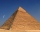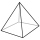# Pythagorean theorem + pyramid - math problems

#### Number of problems found: 77A regular quadrilateral pyramid has a volume of 24 dm3 and a base edge a = 4 dm. Calculate: a/height of the pyramid b/sidewall height c/surface of the pyramid
• 4s pyramidRegular tetrahedral pyramid has a base edge a=17 and collaterally edge length b=32. What is its height?
• The pyramidThe pyramid with a square base is 50 m high and the height of the sidewall is 80 m. Find the endge of the base of the pyramid.
• Height of pyramidThe pyramid ABCDV has edge lengths: AB = 4, AV = 7. What is its height?
• Hexagonal pyramidThe base of the pyramid is a regular hexagon, which can be circumscribed in a circle with a radius of 1 meter. Calculate the volume of a pyramid 2.5 meters high.
• Pyramid heightFind the volume of a regular triangular pyramid with edge length a = 12cm and pyramid height h = 20cm.
• Right pyramidA right pyramid on a base 4 cm square has a slant edge of 6 cm. Calculate the volume of the pyramid.
• Hexagonal pyramidRegular hexagonal pyramid has dimensions: length edge of the base a = 1.8 dm and the height of the pyramid = 2.4 dm. Calculate the surface area and volume of a pyramid.
• Triangular pyramidCalculate the volume of a regular triangular pyramid with edge length a = 12cm and pyramid height v = 20cm.
• Hexagonal pyramidFind the area of a shell of the regular hexagonal pyramid, if you know that its base edge is 5 cm long and the height of this pyramid is 10 cm.
• Pyramid 4sidesCalculate the volume and the surface of a regular quadrangular pyramid when the edge of the base is 4 cm long and the height of the pyramid is 7 cm.We have a regular quadrilateral pyramid with a base edge a = 10 cm and a height v = 7 cm. Calculate 1/base content 2/casing content 3/pyramid surface 4/volume of the pyramidA quadrilateral pyramid, which has a rectangular base with dimensions of 24 cm, 13 cm. The height of the pyramid is 18cm. Calculate 1/the area of the base 2/casing area 3/pyramid surface 4/volume of the pyramid
• Tetrahedral pyramidIt is given a regular tetrahedral pyramid with base edge 6 cm and the height of the pyramid 10 cm. Calculate the length of its side edges.
• Pyramid in cubeIn a cube with an edge 12 dm long, we have an inscribed pyramid with the apex at the center of the cube's upper wall. Calculate the volume and surface area of the pyramid.
• Triangular pyramidWhat is the volume of a regular triangular pyramid with a side 3 cm long?
• Tetrahedral pyramidWhat is the surface of a regular tetrahedral (four-sided) pyramid if the base edge a=16 and height v=16?
• Pentagonal pyramidThe height of a regular pentagonal pyramid is as long as the edge of the base, 20 cm. Calculate the volume and surface area of the pyramid.
• Pyramid four sidesIn a regular tetrahedral pyramid is a body height 38 cm and a wall height 42 cm. Calculate the surface area of the pyramid; the result round to square centimeters.
• PyramidThe pyramid has a base rectangle with a = 6cm, b = 8cm. The side edges are the same and their length = 12.5 cm. Calculate the surface of the pyramid.

Do you have an interesting mathematical word problem that you can't solve it? Submit a math problem, and we can try to solve it.

We will send a solution to your e-mail address. Solved examples are also published here. Please enter the e-mail correctly and check whether you don't have a full mailbox.

Please do not submit problems from current active competitions such as Mathematical Olympiad, correspondence seminars etc...

Pythagorean theorem is the base for the right triangle calculator. Pythagorean theorem - math word problems. Pyramid Problems.